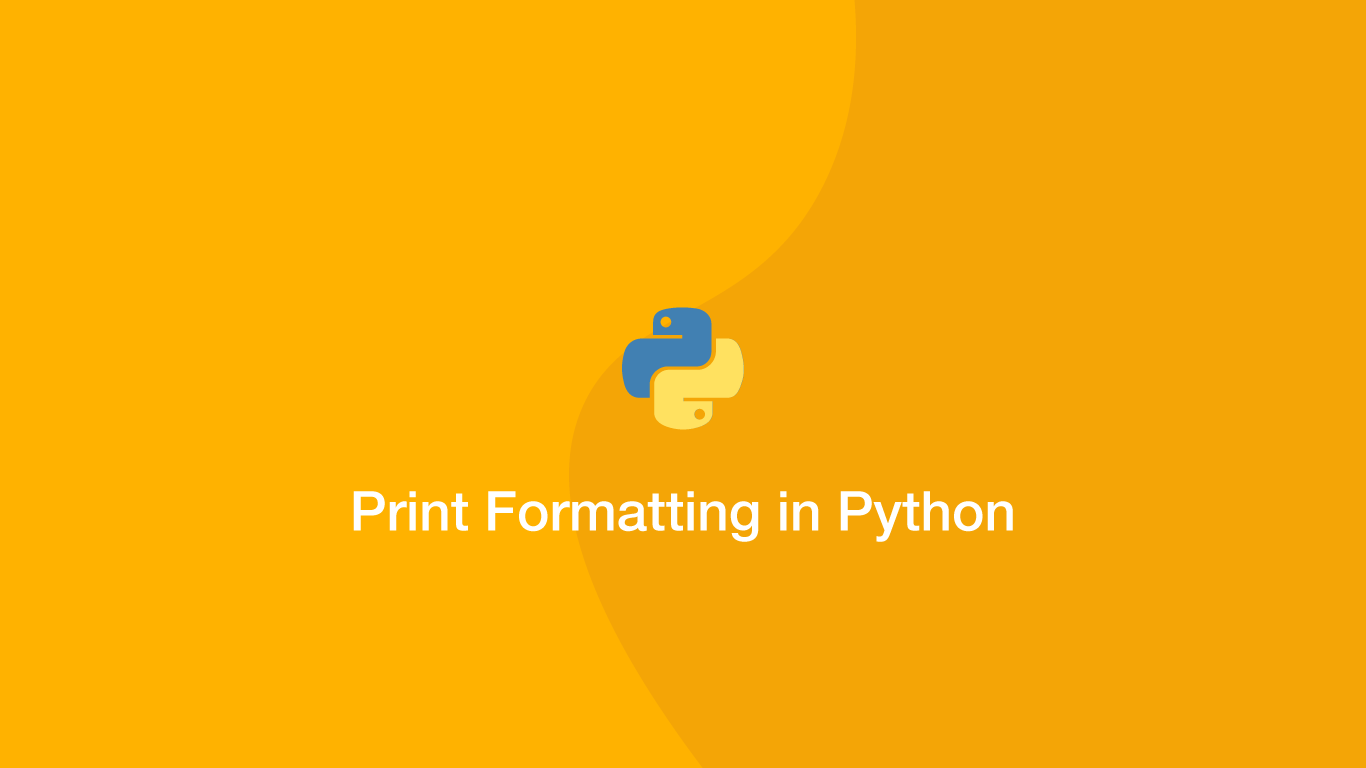# Python Print FormattingIt used to be the case that the `%` (modulo operator) was the only option for formatting strings to print in Python. Now there are a couple more options that make the code more readable, each of which is useful for different situations.

In this tutorial, we will learn how to format the output of a program into a string before printing the data.

## Using the Modulo Operator

Normally, the modulo operator (`%`) is used to divide numbers to the left and right of it. In strings, it is used to fill data from the right side of a modulo operation into a string on the left. Let's have a look an example of this in action.

``````print('%d %s %d' % (2*2, 'is less than', 3*3))
``````
``````4 is less than 9
``````

On the left, we have a string with `%d` and `%s` conversion specifiers, and on the right (after the `%`) we have a list of data that should fill those conversion specifiers. The conversion specifiers on the left must be able to access corresponding data on the right (a counterpart tuple element) or an error will be thrown.

What makes modulo operations powerful in the context of strings is that anything can be put between the conversion specifiers without needing to add anything extra for concatenation.

``````print('%d foo %sblaa bar%d' % (2*2, 'is less than', 3*3))
``````
``````4 foo is less thanblaa bar9
``````

## Using the format() Function

A nicer way to format strings is with the `format()` method. It works in a similar way to the modulo operation, but with a cleaner and more understandable syntax. Let's have a look at an example and then examine what is happening.

``````print('A string that Contains {0} using the {1} method'.format('formatting', 'format()'))
``````
``````A string that Contains formatting using the format() method
``````

Values are extracted from the `format()` method using the index of the argument wrapped in `{}` (curly braces). The argument will have the index of `0`, the second `1` and so on so forth.

Here is another example of the `format()` method this time preparing numbers to be formatted in a string.

``````print('{0} {1} {2} is equal to {3}'.format(2, 'x', 2, 2*2))
``````
``````2 x 2 is equal to 4
``````

## String .format() Nested Replacement Fields

It is possible to create a string with nested data from the `format()` method. This is done by putting the index of the `format()` argument followed by a `:` (colon) inside `{}` (curly braces). Then values can be nested inside.

``````w = 10
print('{1:{0}}'.format(w, 12))
``````
``````12
``````

## Formatted String Literals (F Strings)

Formatted strings were introduced in Python 3.6 and look just like regular strings except with a prepended `f`. F strings accept dynamic data inside `{}` (curly braces) which can contain many different types of expressions. Let's try a few examples of formatted strings to see how they work.

``````x = 10
y = 3

print(f'{x} times {y} = {x*y}')
``````
``````10 times 3 = 30
``````
``````fruit = ['strawberry', 'apricot', 'orange']

print(f'{fruit} or {fruit}?')
``````
``````strawberry or orange?
``````
``````word = 'foo'

print(f'{word.upper()}')
``````
``````FOO
``````

Essentially you can put whatever you need in formatted string literals, except empty expressions and backslashes.

## Center-align the Output

To center align a string, use the `center()` method. This method takes two arguments, the first is the number of characters to use in the centring process and secondly what character to use for the left/right padding.

``````word = 'foo'

print(word.center(30, '#'))
``````
``````#############foo##############
``````

## Left-align the Output

To left-align a string use the `ljust()` method, padding the number of characters to use as the first argument and the padding character in the second.

``````word = 'foo'

print(word.ljust(30, '-'))
``````
``````foo---------------------------
``````

## Right-align the Output

To left-align a string use the `rjust()` method, padding the number of characters to use as the first argument and the padding character in the second.

``````word = 'foo'

print(word.rjust(30, '-'))
``````
``````---------------------------foo
``````

## Conclusion

You now know how to print format strings in Python in a variety of different ways. Personally, I think the f strings offer great readability and powerful functionality at the same time.

#### Related Tutorials### How to use Python datetime to Create and use Dates and Times

September 24, 2020### How to Read CSV Files in Python

October 02, 2020### How to Execute Shell Commands in Python

September 22, 2020### Pretty Print a Dictionary in Python

September 16, 2021### Convert Int to Binary in Python

November 24, 2021June 28, 2021